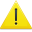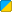# Vector. eqInternal Function: Vector.__eq Vector.__eq is an internal function. This means you will be able to call it, but you really shouldn't.

__eq is a type of overloaded operator. You might have noticed that some objects have a function called __eq. Well, you don't run __eq like this - You run __eq by typing the name of the object, two equal signs (==) and the name of another object.

## Example

For example, you can see if two vectors have the same X, Y, and Z:

Description Compare complimentary X,Y, and Z values and see if they match```  vec1=Vector(5,5,5)*5 vec2=Vector(25,25,25) if vec1==vec2 then Msg("Vector 1 and Vector 2 are equal.\n") end  ``` Vector 1 and Vector 2 are equal - it will say so in the console.